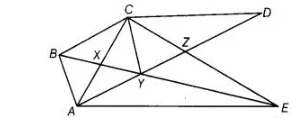# In figure, CD||AE and CY||BA.`
Question:

In figure, CD||AE and CY||BA. Prove that ar (ΔCBX) = ar (ΔAXY).Solution:

Given In figure, CD||AE

and CY || BA

To prove ar (ΔCBX) = ar (ΔAXY) .

Proof We know that, triangles on the same base and between the same parallels are equal . in areas.

Here, ΔABY and ΔABC both lie on the same base AB and between the same parallels CY and BA.

ar (ΔABY) = ar (ΔABC)

=> ar (ABX) + ar (AXY) = ar (ABX) + ar (CBX)

=> ar (AXY) = ar (CBX) [eliminating ar (ABX) from both sides]